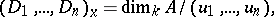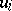# Intersection index (in algebraic geometry)

(diff) ← Older revision | Latest revision (diff) | Newer revision → (diff)

The number of points in the intersection ofdivisors (cf. Divisor) in an-dimensional algebraic variety with allowance for the multiplicities of these points. More precisely, letbe an-dimensional non-singular algebraic variety over a field, and letbe effective divisors inthat intersect in a finite number of points. The local index (or multiplicity) of intersection of these divisors at a pointis the integerwhereis the local equation for the divisorin the local ring. In the complex case, the local index coincides with the residue of the form, and also with the degree of the germ of the mapping (cf. Degree of a mapping)The global intersection indexis the sum of the local indices over all points of the intersection. If this intersection is not empty, then.